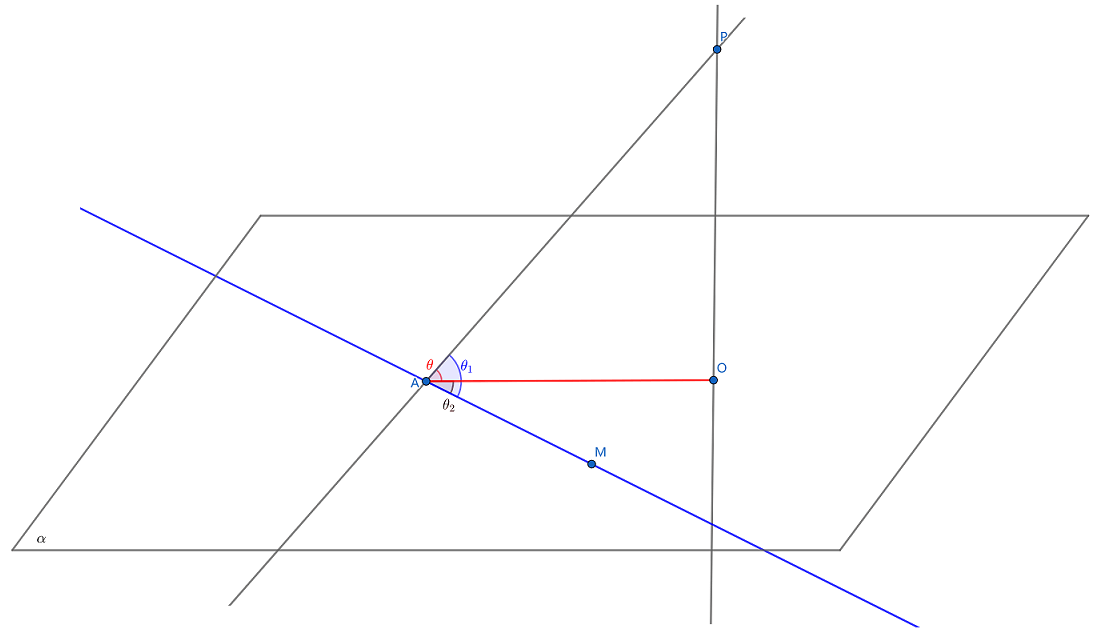安卓手机扫描二维码安装App

A. ﻿$\cos \theta = \cos \theta _1 \cdot \cos \theta _2$﻿

B. ﻿$\cos \theta _1 =\cos \theta \cdot \cos \theta _2$﻿

C. ﻿$\cos \theta _2 = \cos \theta \cdot \cos \theta _1$﻿苹果手机扫描二维码安装App